Disclosure: This post may contain affiliate links, meaning when you click the links and make a purchase, we receive a commission.

# How to divide columns in Excel (Top 8 ways)

In this article, I will show how to divide columns in Excel. I will show several ways. Choose one that best suits your job.

So, let’s start.

## Division Symbol in Excel

In mathematics, to divide two numbers we use an obelus symbol (÷).

15 ÷ 5 = 3

But in Excel, we use the forward-slash (/) to divide two numbers.

15/5 = 3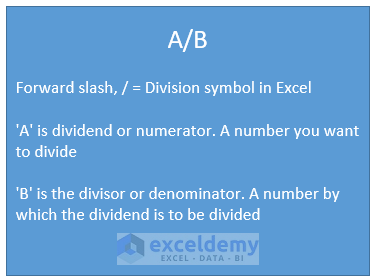In Excel, we write a division expression like the above image, where:

• ‘A’ is the dividend or numerator – a number you want to divide by another number (divisor).
• ‘B’ is the divisor or denominator – a number by which you want to divide another number (dividend).

## How to divide numbers in Excel

Here are some examples of division in Excel.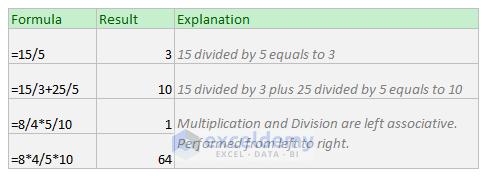To know more about operator precedence and associativity in Excel, read this article: What is the Order & Precedence of Operations in Excel?

## 1) How to divide a cell by another cell/number in Excel

Dividing a cell by another cell or number is the same as dividing two numbers in Excel.

In the place of numbers, we just use cell references. Cell references can be relative, absolute, or mixed.

Here are some examples of dividing cells by another cell or number (image below).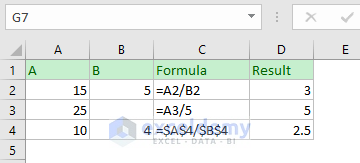• Dividing a cell by another cell: A2/B2
• Dividing a cell by value: A3/5
• Dividing a cell by another cell: \$A\$4/\$B\$4 (cell reference is absolute)

## 2) How to divide one column by another column in Excel (copying formula)

We want to divide the values of column A by the values of column B.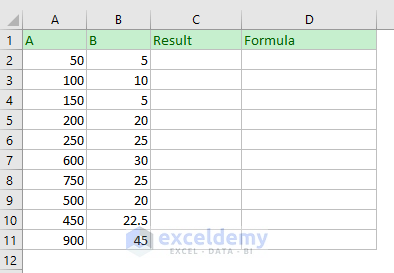• In the cell C2, I type formula =A2/B2.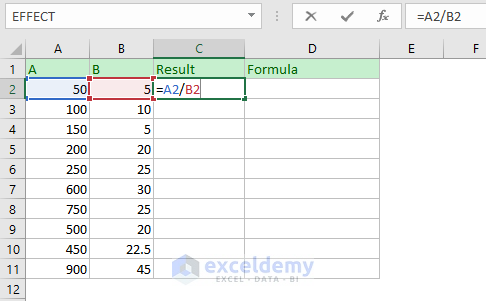• Press Enter. The formula outputs 10 as 50 divided by 5 returns 10. I select the cell C2 And copy the formula in the C2 to other cells below holding and dragging the Excel’s autofill handle tool. Or you can just double click on the autofill handle tool.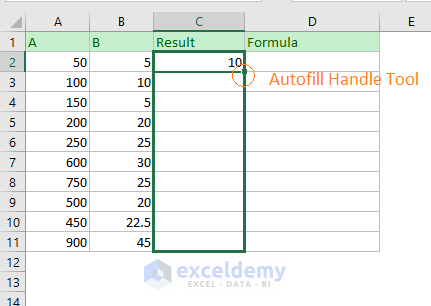• And here is the result. On the right side, the formula is shown.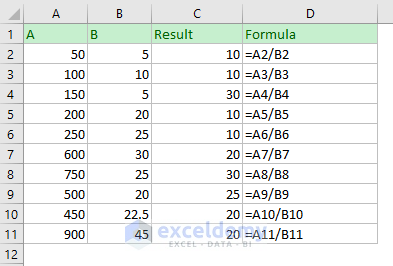## 3) How to divide one column by another one (using Excel’s Array formula)

Let’s divide a column by another one using Excel’s array formula. Most general Excel users fear of Excel’s Array Formula. If you’re one of them, read this article: Excel Array Formula Basic: What is an Array in Excel.

How an array formula can benefit us in this case?

If you want to keep safe your Excel formula to be altered or deleted by your users, you can use the array formula.

Let me show how you could perform the above calculations using an array formula.

• Select the cell range C2 to C11 and I type this formula: =A2:A11/B2: B11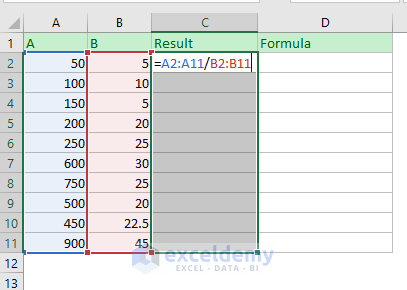• Now press CTRL + SHIFT + ENTER (I remember it with the short form CSE) simultaneously. This is the way to make an array formula in Excel. You see the results.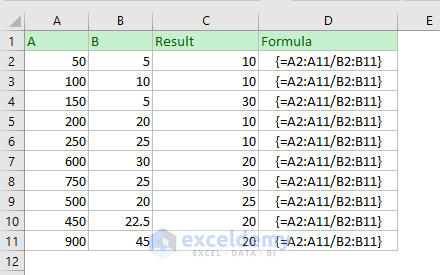A notable thing is: all the cells (C2: C11) are holding the same formula. So, you cannot change the formula of a single cell. To change the formula, you have to select all the cells and then you can edit or delete the formula. After Editing or Deleting the formula, you have to press simultaneously CTRL + SHIFT + ENTER again.

## 4) How to divide a column by a number (using formula)

### Process 1: Using the hard-coded number

Suppose you want to divide the values of a column by a specific number 10 (it can be any).

• In the cell B2, I write this formula: =A2/10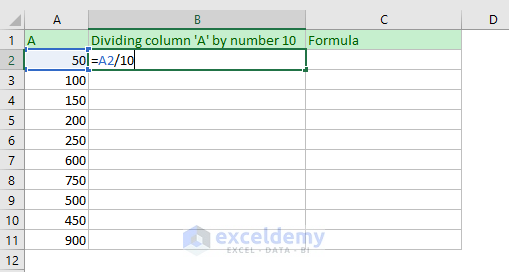• Press Enter and then use the formula for other cells below. Here is the output.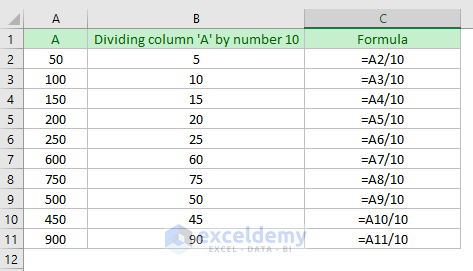So, the values of column A is divided by a specific number 10.

### Process 2: Dynamic method

Maybe one day, you might want to divide those numbers by using another number, say 5. It is not wise to edit the formula to get results.

We can use this method.

• In cell B5, I use this formula: =A5/\$B\$2. In cell B2, I have placed the number that will be used to divide the values of column A.

Observe the formula. You see I have made the B2 cell reference absolute as I don’t want it to be changed when I shall use the formula for other cells in the column.• Now press Enter and use the formula for other cells in the column. Here are the results.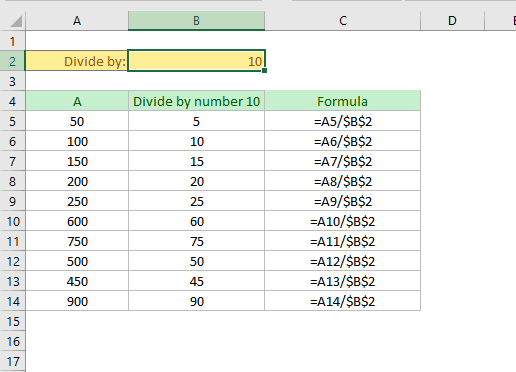## 5) Dividing a column by a number (using Paste Special)

In this method, you can divide a column by a specific number without using an Excel formula.

We shall use Excel’s Paste Special feature.

• We place the divisor in a cell. Suppose in our case, the divisor is in cell B2. Select the cell and copy the value of the cell (keyboard shortcut CTRL + C) command.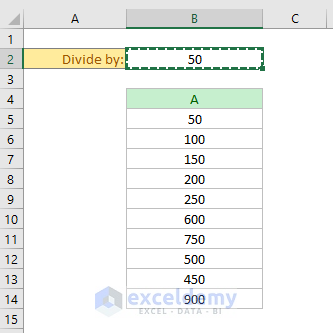• Now I select the numbers under column A -> Right click on my mouse. A menu will appear -> From the menu, click on the Paste Special

Keyboard shortcut to open the Paste Special dialog box: CTRL + ALT + V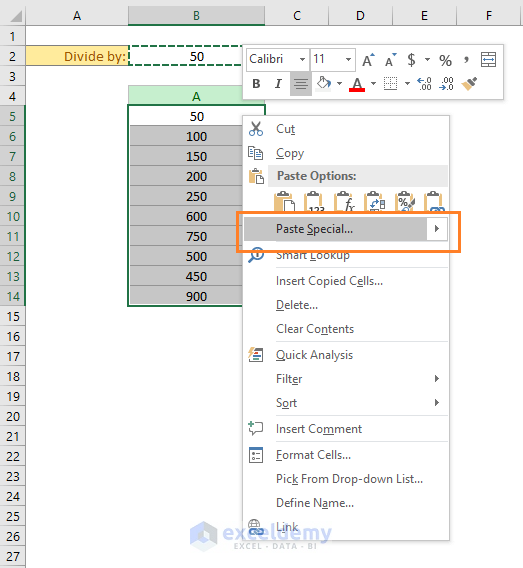• Paste Special dialog box will appear. In this dialog box, select the Divide option (lower right corner of the dialog box). Finally, click on the OK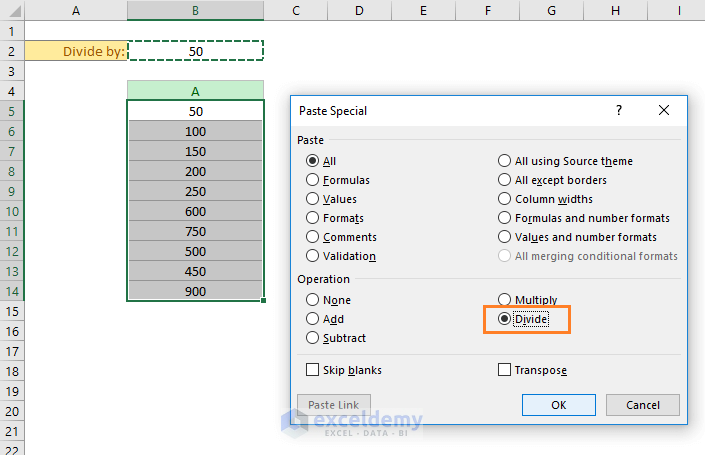• Here is the result.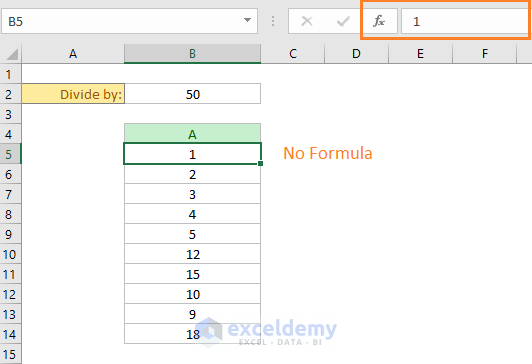All the numbers are replaced by new values (divided by 50). No formulas are seen in the cells.

## 6) How to divide a column using Excel QUOTIENT function

Excel’s Quotient function returns only the integer portion of a division.

Here is the syntax of Excel’s QUOTIENT function: QUOTIENT(numerator, denominator)

Check out the differences between the result done with the general Excel formula and using the QUOTIENT function.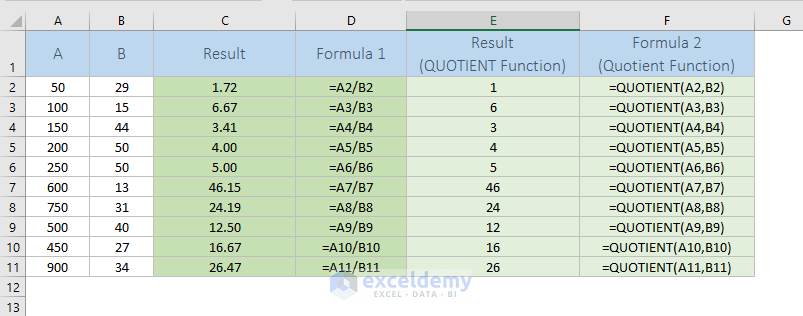## 7) How to divide a column by percentage

You know 25% = 25/100 = 0.25

So, dividing a column by percentage is actually the same as dividing a column by a number.

Here are some examples of dividing a column by percentages.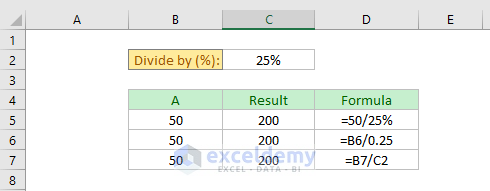• =50/25% = 200
• =B6/0.25 = 200
• =B7/C2 = 200

## 8) Handling #DIV/0! Error while dividing (using IFERROR function)

You cannot divide a number by zero. This is not allowed in mathematics.

When you try to divide a number by zero, Excel shows #DIV/0! Error.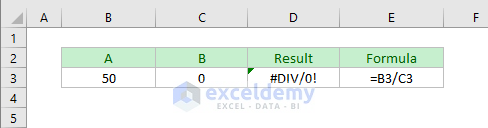We can handle this error in two ways:

• Using Excel IFERROR function
• And using Excel IF function

### Handling #DIV/0! Error using IFERROR function

Here is the syntax of Excel’s IFERROR function: IFERROR (value, value_if_error)

See how I have used the IFERROR function to handle the #DIV/0! Error in Excel.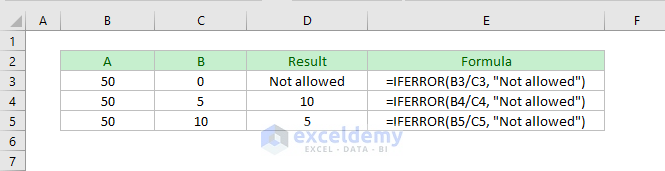I have used this formula in the cell D2: `=IFERROR(B3/C3, "Not allowed")`

### Handling #DIV/0! Error using the IF function

Here is the syntax of Excel’s IF function: IF(logical_test, [value_if_true], [value_if_false])

Instead of using the IFERROR function, you can also use the IF function to handle the #DIV/0! Error (following image).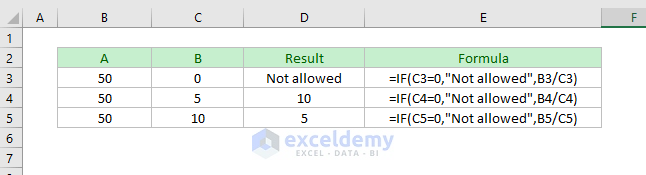In the cell D2, I have used this formula: `=IF(C3=0,"Not allowed",B3/C3)`

Then I copy-pasted this formula for other cells in the column.

## Conclusion

These are my ways of dividing columns in Excel. Do you know any other methods? I am eager to know. Please let me know in the comment box.

Hello!
Welcome to my Excel blog! It took me some time to be a fan of Excel. But now I am a die-hard fan of MS Excel. I learn new ways of doing things with Excel and share here. Not only how to guide on Excel, but you will get also topics on Finance, Statistics, Data Analysis, and BI. Stay tuned!

1. ReplyPrithviraj Dec 6, 2018 at 5:18 PM

It’s really amazing sir , you are great.

• ReplyDec 6, 2018 at 5:22 PM

2. ReplyAndrew Apr 11, 2019 at 9:07 PM

Thank you for introducing me to the word “obelus” and the quotient function.

• ReplyApr 11, 2019 at 9:42 PM

You’re welcome, Andrew 🙂

3. ReplySarabjeet Singh Apr 15, 2019 at 12:08 AM

Hi,

The description is very well organized and thanks to the developer for provising such knowledge.

• ReplyApr 15, 2019 at 1:32 PM

Thanks, Sarabjeet Singh, for your feedback!